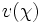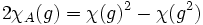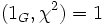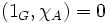# Indicator theorem

## Statement

The following information about an irreducible linear representation over complex numbers can be garnered from the Frobenius-Schur indicator of its character$\chi$ (denoted$v(\chi)$) (viz, its inner product with the indicator character):

•$v(\chi) = 0$ if and only if$\chi$ is not real-valued
•$v(\chi) = 1$ if and only if$\chi$ is the character of a real representation
•$v(\chi)=-1$ if and only if$\chi$ is real-valued but does not arise as the character of a real representation

## Proof

Let$\rho:G \to GL(V)$ be the linear representation giving the character$\chi$. Denote by$Sym^2(\rho)$ the corresponding representation on$Sym^2(V)$ and by$Alt^2(\rho)$ the corresponding representation on$Alt^2(V)$. Let$\chi_A$ be the character of$Alt^2(\rho)$.

Then, by some elementary computations:$2\chi_A(g) = \chi(g)^2 - \chi(g^2)$

From this it follows that:$v(\chi) = (1_G, \chi^2 - 2\chi_A) = (1_G,\chi^2) - 2(1_G,\chi_A)$

Now since$Alt^2(\rho)$ is a direct summand of$\rho \otimes \rho$, we must have$1_G,\chi_A) \le (1_G,\chi^2)$. But if$\chi$ is not real-valued, then$(1_G,\chi^2) = 0$ so$v(\chi) = 0$ and if$\chi$ is real-valued, then$(1_G,\chi^2) = 1$. Now two cases arise:

•$(1_G,\chi_A) = 1$ and hence$v(\chi) = -1$. This happens only if$\chi$ is not the character of a real representation.
•$(1_G,\chi_A) = 0$ and hence$v(\chi) = 1$. This happens only if$\chi$ is the character of a real representation.

The idea behind proving the latter distinction is to relate this with the existence of a group-invariant symmetric bilinear form.